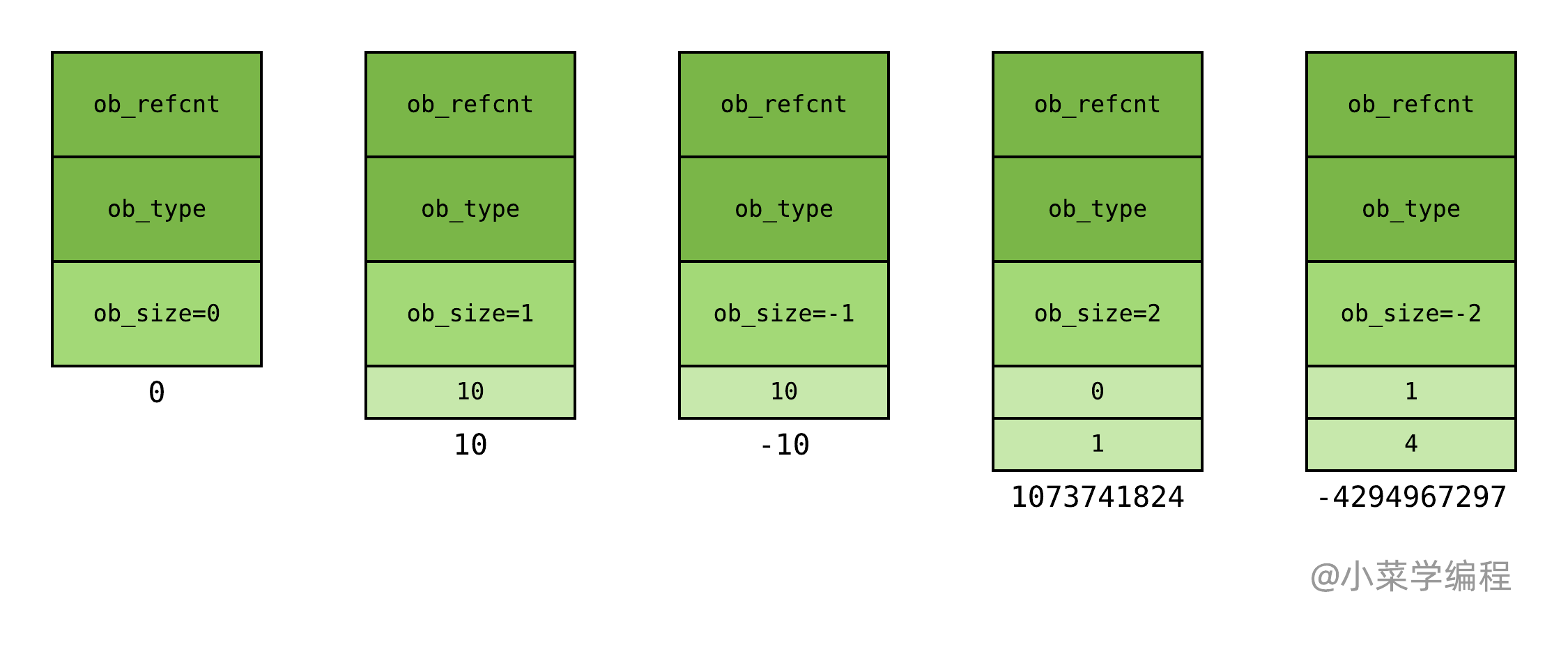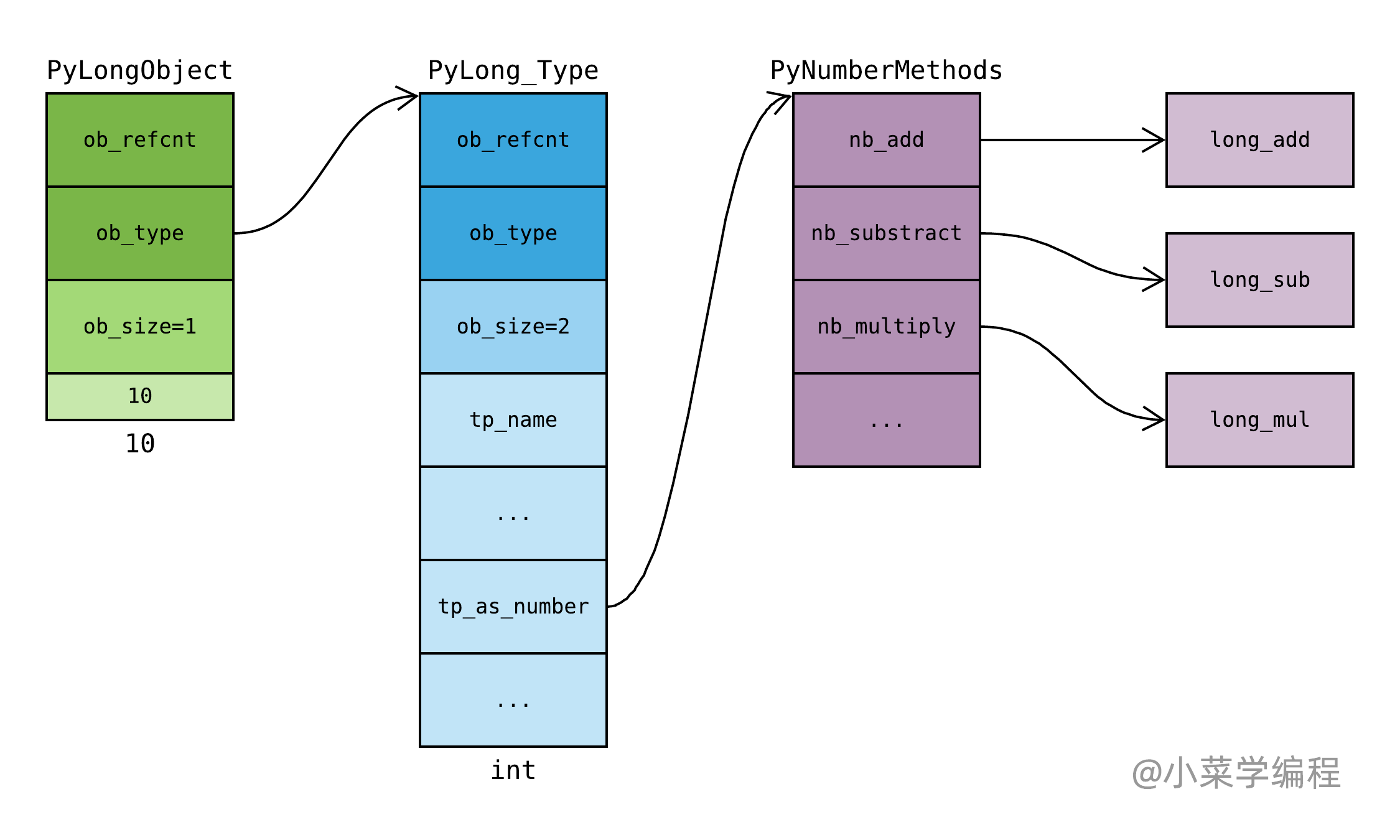# int 源码解析：如何实现大整数运算？• ob_digitC 整数数组，用于存储被保存整数的 绝对值 ；
• ob_size变长对象 关键字段，维护数组长度以及被保存整数的 符号 ；

Python 整数对象通过串联多个 C 整数类型，实现大整数的表示。整数对象内部包含一个 C 整数数组，数组长度与对象表示的数值大小相关，因此整数对象也是 变长对象 。## 数学运算概述

  1 2 3 4 5 6 7 8 9 10 11 12 13 14 15 16  PyTypeObject PyLong_Type = { PyVarObject_HEAD_INIT(&PyType_Type, 0) "int", /* tp_name */ offsetof(PyLongObject, ob_digit), /* tp_basicsize */ sizeof(digit), /* tp_itemsize */ long_dealloc, /* tp_dealloc */ //... &long_as_number, /* tp_as_number */ //... long_new, /* tp_new */ PyObject_Del, /* tp_free */ }; 

  1 2 3 4 5 6 7 8 9 10 11 12 13 14 15 16 17 18 19 20  static PyNumberMethods long_as_number = { (binaryfunc)long_add, /*nb_add*/ (binaryfunc)long_sub, /*nb_subtract*/ (binaryfunc)long_mul, /*nb_multiply*/ long_mod, /*nb_remainder*/ long_divmod, /*nb_divmod*/ long_pow, /*nb_power*/ (unaryfunc)long_neg, /*nb_negative*/ (unaryfunc)long_long, /*tp_positive*/ (unaryfunc)long_abs, /*tp_absolute*/ (inquiry)long_bool, /*tp_bool*/ (unaryfunc)long_invert, /*nb_invert*/ long_lshift, /*nb_lshift*/ (binaryfunc)long_rshift, /*nb_rshift*/ long_and, /*nb_and*/ long_xor, /*nb_xor*/ long_or, /*nb_or*/ long_long, /*nb_int*/ // ... };## 加法

  1 2 3 4 5 6 7 8 9 10 11 12 13 14 15 16 17 18 19 20 21 22 23 24 25 26 27 28 29  static PyObject * long_add(PyLongObject *a, PyLongObject *b) { PyLongObject *z; CHECK_BINOP(a, b); if (Py_ABS(Py_SIZE(a)) <= 1 && Py_ABS(Py_SIZE(b)) <= 1) { return PyLong_FromLong(MEDIUM_VALUE(a) + MEDIUM_VALUE(b)); } if (Py_SIZE(a) < 0) { if (Py_SIZE(b) < 0) { z = x_add(a, b); if (z != NULL) { assert(Py_REFCNT(z) == 1); Py_SIZE(z) = -(Py_SIZE(z)); } } else z = x_sub(b, a); } else { if (Py_SIZE(b) < 0) z = x_sub(a, b); else z = x_add(a, b); } return (PyObject *)z; }